# Pressure Force Area

GCSELevel 4-5AQACambridge iGCSEEdexcelEdexcel iGCSEOCR

## Pressure Force Area

Pressure is a measure of how much force is applied over a given area of an object, so it is calculated by dividing the amount of force being applied by the area over which it is being applied.

Make sure you are happy with the following topics before continuing.

Level 4-5GCSEAQAEdexcelOCRCambridge iGCSEEdexcel iGCSE

## Pressure Force Area – Formula

Pressure, force and area are all related by the formula:

$p = \dfrac{F}{A}$

where $p$ is the pressure, $F$ is force, and $A$ is area. You can rearrange this formula to find the other two, for example, if we multiply both sides of the equation by $A$, then swap the left-hand side and right-hand side, we get.

$F = p \times A$

So, we we can calculate the force by multiplying the pressure by the area.

Since area is measured in square metres (m$^2$), and force is measured in Newtons (N), the standard units for pressure are Newtons per square metre (N/m$^2$). These are compound units (for more information, see Conversions revision).

Level 4-5GCSEAQAEdexcelOCRCambridge iGCSEEdexcel iGCSE

## Pressure Force Area – Formula Triangle

A quick way of remembering how to calculate one of either pressure, force or area using the other two is to use the triangles below.

The horizontal line means divide and the $\times$ symbol means multiply. We then:

We then cover up the one we want to find (represented by a red circle) and substitute values into the formula for the two remaining quantities in the triangle.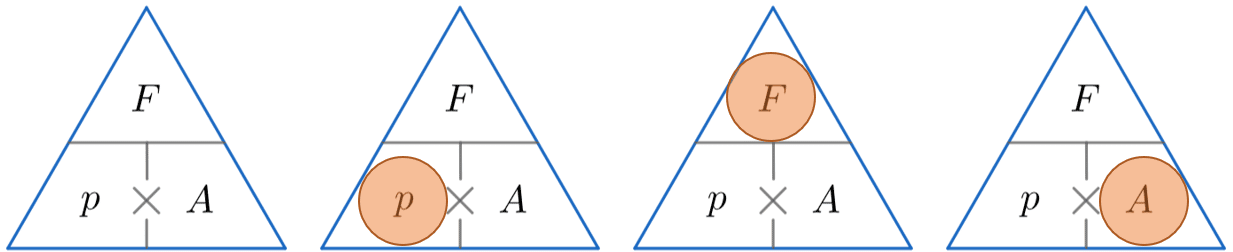$p = \dfrac{F}{A} \,\,\,\,\,\,\,$ $\,\,\,\,\,\,\,\,\,\, F = p\times A\,\,\,\,\,\,\,\,\,$ $\,\,\,\,\,\,\,\,\, A = \dfrac{F}{p}$

Level 4-5GCSEAQAEdexcelOCRCambridge iGCSEEdexcel iGCSE

## Example 1: Calculating Pressure

A force of $150$ N is being applied over an area measuring $0.5$ m$^2$. Calculate the pressure on the object ensuring you give the correct units.

[2 marks]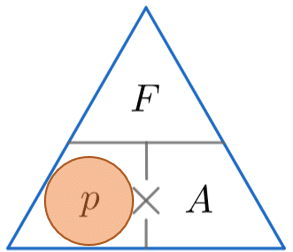We’re looking for pressure, so constructing the triangle and covering up the $p$, we must divide the force by the area. So

$\text{Pressure } = 150 \div 0.5 = 300$ N/m$^2$

Level 4-5GCSEAQAEdexcelOCRCambridge iGCSEEdexcel iGCSE

## Example 2: Calculating Force

A woman is applying $300$ N/m$^2$ of pressure onto a door with her hand. Her hand has area $0.02$ m$^2$. Work out the force being applied.

[2 marks]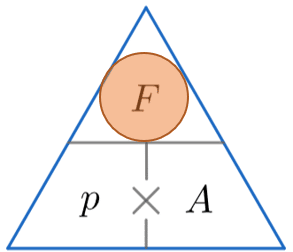We’re looking for force, so constructing the triangle and covering up the $F$, we must multiply the pressure by the area. So

$\text{Force } = 300 \times 0.02 = 6$ N

Level 4-5GCSEAQAEdexcelOCRCambridge iGCSEEdexcel iGCSE

## Example 3: Calculating Area

Pressure of $150$ N/m$^2$ is experienced when a force of $2$ kN is applied. Calculate the area over which the force is applied to obtain the pressure stated.

[2 marks]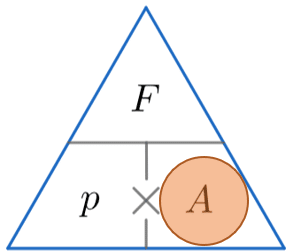Now, before we do any calculations, we need to make sure our units are in the same form. In this example we need to convert kilo-newtons into newtons by multiplying by $1000$. This gives us $2000$ N.

We’re looking for area, so constructing the triangle and covering up the $A$, we must divide the force by the pressure. So

$\text{Area } = 2000 \div 150 = 13.3$ m$^2$

Level 4-5GCSEAQAEdexcelOCRCambridge iGCSEEdexcel iGCSE

## Pressure Force Area Example Questions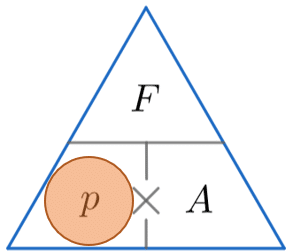We are calculating the pressure, so  by covering up $p$, we can see from the triangle above that we have to divide $F$ by $A$.

Before we can do this calculation however, we need to work out the area of the square.  Since the square has  a side length of $3$ m, the area can be calculated as follows:

$3$ m $\times \, 3$ m $= 9$ m$^2$

Now that we have the area and the force, we can calculate the pressure by substituting the values for the area and the force into the pressure equation as follows:

$\text{pressure }=\dfrac{F}{A}=\dfrac{185.6}{9}=20.6222...=20.6$ N/m$^2$ ($3$ sf).

The units must be N/m$^2$ since the units used in the question are N and m$^2$.Gold Standard EducationWe are calculating the area, so by covering up $A$, we can see from the triangle above that we have to divide $F$ by $p$.  So, by substituting the known values for the force and the pressure into the equation, we can calculate the area as follows:

$\text{Area }=\dfrac{F}{p}=\dfrac{740}{2312.5}=0.32latex] m[latex]^2$

The unit must be m$^2$ since the units used in the question are N/m$^2$.Gold Standard Education

In this question, we are calculating the force, so we will need to rearrange the pressure / force  / area equation.

Since

$\text{pressure = force} \div \text{ area}$

then

$\text{force = pressure} \times \text{ area}$

By substituting the values for the pressure and the area into the formula above, we can calculate the force as follows:

$\text{Force } = 16$ m$^2 \times \, 2480$ N/m$^2 = 39,680$ NGold Standard Education

This may seem like an impossible question without sufficient information to go by at first glance.  We have been given the pressure as well as the weight (force) of the cylinder.  Since we know both the force and the pressure, the only thing we are able to calculate at this stage is the area of the circular cylinder face.

Since

$\text{pressure = force} \div \text{ area}$

then

$\text{area = force} \div \text{ pressure}$

By substituting the values for the force and the pressure into the formula above, we can calculate the area as follows:

$4872$ N $\div \, 812$ N/m$^2 = 6$ m$^2$

We now know that the area of the circular face on which the cylinder is resting has an area of $6$ m$^2$, and from this information we need to work out the diameter of the cylinder / circle.  In this question, we therefore need to apply some basic knowledge about the circle areas.

The formula for the area of a circle is $\pi r²$.

However, we know the area and want a formula for the radius, so we will need to rearrange this formula.

First of all, we can both sides by $\pi$:

$\dfrac{a}{π} = r^2$

If we now take the square root of both sides, we have a formula for the radius:

$\sqrt \dfrac{a}{π} = r$

Since we know the area, all we need to do is divide the area by $\pi$, and square root the answer.

$6 \div \pi = 1.9099$

$\sqrt {1.9099} = 1.38$ m

If the radius of the circle is $1.38$m, then the diameter will be double this:

$\text{Diameter} = 2 \times 1.38 = 2.76$ mGold Standard Education

This is another of these questions that might seem impossible initially, but there is sufficient information in the question to solve it.  Like many maths problems, if you don’t have a clear strategy, just do any calculations you can, as they may lead somewhere useful!Gold Standard Education

## Pressure Force Area Worksheet and Example Questions

### (NEW) Pressure Force Area Exam Style Questions - MME

Level 4-5GCSENewOfficial MME

## Pressure Force Area Drill Questions

### Density and Pressure - Drill Questions

Level 4-5GCSEGold Standard Education

£19.99 /month. Cancel anytime

Level 6-7GCSE

Level 4-5GCSE